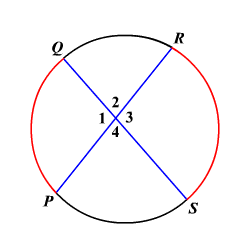# Angles of Intersecting Chords Theorem

If two chords intersect inside a circle, then the measure of the angle formed is one half the sum of the measure of the arcs intercepted by the angle and its vertical angle.In the circle, the two chords $\overline{PR}$ and $\overline{QS}$ intersect inside the circle.

$m\angle 1=\frac{1}{2}\left(m\stackrel{⌢}{PQ}+m\stackrel{⌢}{RS}\right)$ and $m\angle 2=\frac{1}{2}\left(m\stackrel{⌢}{QR}+m\stackrel{⌢}{PS}\right)$

Since vertical angles are congruent, $m\angle 1=m\angle 3$ and $m\angle 2=m\angle 4$.

Example:

In the circle shown, if $m\stackrel{⌢}{PQ}=92°$ and $m\stackrel{⌢}{RS}=110°$, then find $m\angle 3$.

Substitute.

$\begin{array}{l}m\angle 3=\frac{1}{2}\left(m\stackrel{⌢}{PQ}+m\stackrel{⌢}{RS}\right)\\ \text{\hspace{0.17em}}\text{\hspace{0.17em}}\text{\hspace{0.17em}}\text{\hspace{0.17em}}\text{\hspace{0.17em}}\text{\hspace{0.17em}}\text{\hspace{0.17em}}\text{\hspace{0.17em}}\text{\hspace{0.17em}}\text{\hspace{0.17em}}\text{\hspace{0.17em}}=\frac{1}{2}\left(92°+110°\right)\\ \text{\hspace{0.17em}}\text{\hspace{0.17em}}\text{\hspace{0.17em}}\text{\hspace{0.17em}}\text{\hspace{0.17em}}\text{\hspace{0.17em}}\text{\hspace{0.17em}}\text{\hspace{0.17em}}\text{\hspace{0.17em}}\text{\hspace{0.17em}}\text{\hspace{0.17em}}=\frac{1}{2}\left(202°\right)\\ \text{\hspace{0.17em}}\text{\hspace{0.17em}}\text{\hspace{0.17em}}\text{\hspace{0.17em}}\text{\hspace{0.17em}}\text{\hspace{0.17em}}\text{\hspace{0.17em}}\text{\hspace{0.17em}}\text{\hspace{0.17em}}\text{\hspace{0.17em}}\text{\hspace{0.17em}}=101°\end{array}$

Therefore, $m\angle 3=101°$.